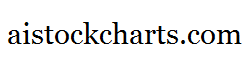Members Area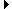Sign Up | Log In
 Free Level 2 QuotesStock AnalysisCorrelation Trading PlatformCorrelationsMembership Benefits

GAP
 Lookup a different indicator.

 Indicator Usage Description Example gap gap[x,y,z,n] , where x {fu, fd, pu, pd, uu, ud} and y & z are optional positive integers <= 100% representing the minimum and maximum percentage price gap change. n is optional and represents the number of days ago the result is computed (n=0 is the default value and represents the current end of day, n=1 is the previous end of day, etc.) Returns a 1 (logical TRUE) if the price gap from n days ago matches x, fu=Full Up Gap, fd=Full Down Gap, pu=Partial Up Gap, pd=Partial Down Gap, uu=Unfilled Up Gap, ud=Unfilled Down Gap (gap[fu,1])Screens for full up gaps where the opening price is > 1% higher than the previous day high price.

Introduction:

The 'gap' indicator is used in indicator formula construction to narrow the stock pre-screener results to include only those stocks that have a stock price gap restricted to the parameters set for the gap indicator.

Example:

The chart of Intel (INTC) below highlights days when there were full up gaps where the opening price is > 1% higher than the previous day high price.

 Chart of Intel (INTC) highlighting days where the slope of the 25-day SMA was positive and the stock price closed above the 25-day SMA.

INDICATORS IN OUR DATABASE THAT INCLUDE THE GAP INDICATOR...

Click here to view all indicators that include the indicator 'gap' (sorted by AISCORE)

 (gap[fu])AISCORE: 918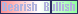Indicator ID: 070712193828(gap[fd])AISCORE: 3257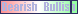Indicator ID: 070712200345(gap[pd])AISCORE: 2056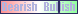Indicator ID: 070712200415(gap[pu])AISCORE: 2249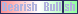Indicator ID: 070712200457(gap[uu])AISCORE: 1250Indicator ID: 070712200516 More ->